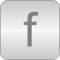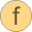# General

Package with utility functions# Package ContentsCubicInterpolation_DPCubicInterpolation_MFLOWLambertW Closed approximation of Lambert's w function for solving f(x) = x exp(x) for xLambertWIter Iterative form of Lambert's w function for solving f(x) = x exp(x) for xPrandtlNumber calculation of Prandtl numberReynoldsNumber calculation of Reynolds numberSmoothPower Limiting the derivative of function y = if x>=0 then x^pow else -(-x)^powSmoothPower_der The derivative of function SmoothPowerStepsmoother Continuous interpolation for xStepsmoother_der Derivative of function Stepsmoother